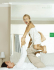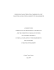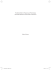# Specifying a Ferrite for EMI Suppression

## Transcription

Specifying a Ferrite for EMI Suppression
```on
EMC Components
Specifying a Ferrite
for EMI Suppression
by Carole U. Parker, Fair-Rite Products
50 Conformity JUNE 2008
O
ur past article (see “How to Choose Ferrite
Components for EMI Suppression,” Conformity, June
2002) was intended to help design engineers optimize
the performance of ferrite materials by analyzing the effects
of frequency, field strength, temperature and core geometry.
In our ideal world, safety (including effect on environment),
quality and performance are paramount. However, the cost of
the final component (which includes the ferrite) has, in many
cases, become the deciding factor.
for alternative ferrite materials as a means to reduce cost.
A Review of Ferrite Applications
The following are three major applications for soft ferrite:
1. Low signal level
2. Power
3. EMI
The required intrinsic material characteristics and core
geometry are dictated by each specific application. The
intrinsic characteristics controlling the performance of low
signal level applications are permeability (particularly with
temperature), low core loss, and good magnetic stability with
time and temperature. Applications include high Q inductors,
common mode inductors, wideband,
matching and pulse transformers, antenna
elements for radios and both active
and passive transponders. For power
applications, the desirable characteristics
are high flux density and low losses at
the operating frequency and temperature.
Applications include switchmode power
supplies, magnetic amplifiers, dc-dc
converters, power filters, ignition coils,
and transformers for battery charging of
electrical vehicles.
The third, and most common use, is when ferrite cores are
used alone on component leads or in board level circuitry.
In this application, the ferrite core prevents any parasitic
oscillations and/or attenuates unwanted signal pickup or
transmission that might travel along component leads or
interconnected wires, traces, or cables.
In both the second and third applications, the ferrite core
suppresses the conducted EMI by eliminating or greatly
reducing the high frequency currents emanating from the EMI
source. The introduction of the ferrite provides a sufficiently
high frequency impedance that results in the suppression of
the high frequency currents. Theoretically, the ideal ferrite
would provide a high impedance at EMI frequencies, and
zero impedance at all other frequencies. In reality, ferrite
suppresser cores provide a frequency-dependent impedance,
that is, low at frequencies below 1 MHz, and (depending upon
the ferrite material) the maximum impedance that can be
obtained between 10 MHz to 500 MHz.
Complex Permeability
As is consistent with electrical engineering principles in
which alternating voltages and currents are denoted by
complex parameters, so the permeability of a material can be
represented as a complex parameter consisting of a real and an
imaginary part. This is evidenced at high frequencies where
the permeability separates into two components. The real
The intrinsic characteristic that most
influences the performance of soft
ferrite in suppression applications is the
complex permeability, which is directly
proportional to the core’s impedance.
There are three ways to use ferrites
as suppressors of unwanted signals,
conducted or radiated. The first, and least
common, is as actual shields where ferrite
is used to isolate a conductor, component
or circuit, from an environment of
the second application, the ferrite is used
with a capacitive element to create a low
pass filter that is inductance-capacitance
at low frequencies and dissipative at
higher frequencies.
JUNE 2008 Conformity 51
component (µ') represents the reactive portion, and is in phase1
with the alternating magnetic field, whereas the imaginary
component (µ") represents the losses, and is out of phase with
the alternating magnetic field.
materials. Material type 73 is a manganese zinc ferrite with an
initial permeability of 2500. Material type 43 is a nickel zinc
ferrite with an initial permeability of 850. Material type 61 is a
nickel-zinc ferrite with an initial permeability of 125.
These may be expressed as series components (µs' µs" ) or
parallel components (µp' µp"). The graphs in Figures 1, 2
and 3 show the series components of the complex initial
permeability as a function of frequency for three ferrite
Concentrating on Figure 3, the series components of 61 type
material, we see that the real part of the permeability, µs',
remains constant with increasing frequency until a critical
frequency is reached and then decreases rapidly. The losses, or
µs", rise, then peak as µs' falls. This decrease in µs' is due to the
onset of ferrimagnetic resonance2. It should be noted that the
higher the permeability, the lower the frequency at which this
occurs. This inverse relationship was first observed by Snoek
and given the following formula:
ƒres =
γ Msat
Hz
3� (µi - 1)
Equation 1
Where:
ƒres = frequency at which µs" is maximum
γ = gyromagnetic ratio=0.22 x 106 A-1 m
µi = initial permeability
Msat = 250-350 Am-1
Figure 1
This same Equation can be approximated by:
ƒres = Bsat/µi MHz Equation 2
Since ferrite cores used in low signal level and power
applications are concerned with magnetic parameters below
this frequency, rarely does the ferrite manufacturer publish
data for permeability and/or losses at higher frequencies.
However, higher frequency data is essential when specifying
ferrite cores used in the suppression of EMI.
Relationship Between Complex Permeability and Impedance
Figure 2
The characteristic that is specified by most ferrite
manufacturers for components used in EMI suppression
is impedance. Impedance is easily measured on readily
available commercial analyzers with direct digital readouts.
Unfortunately, impedance is usually specified at particular
frequencies and is the scalar quantity representing the
magnitude of the complex impedance vector. Although this
information is valuable, it is often not sufficient, especially
when modeling the ferrite’s circuit performance. In order to
accomplish this, the impedance value and phase angle for
the components, or the complex permeability for the specific
material, must be available.
But even before beginning to model the performance of a
ferrite component in a circuit, the designer should know the
following:
•• The frequency of unwanted signals;
Figure 3
52 Conformity JUNE 2008
•• The source of the EMI (radiated/conducted);
•• Operating conditions (environment);
•• If high resistivity is required because of multiple turns,
conductor pins in a connector filter plate or position in the
circuit;
•• The circuit impedance, source and load;
At low frequencies, the impedance of the component is
primarily the inductive reactance. As frequency increases,
the inductance decreases at the same time the losses increase
and the total impedance increases. Figure 4 is a typical curve
of XL, Rs and Z vs. frequency for our a medium permeability
material.
•• How much attenuation is required;
Knowing that, the magnetic quality factor:
•• The allowable space on the board.
Q = µ'/µ" = ωLs/Rs Equation 8
The design engineer can then compare materials at the
relevant frequencies for the complex permeability, heeding
effects of temperature and field strength. After this, core
geometry can be selected, from which the inductive reactance
and series resistance can be defined.
Then, the inductive reactance is made directly proportional to
the real part of the complex permeability by Lo, the air core
inductance:
The Equations
The impedance of a ferrite core in terms of permeability is
given by:
The loss resistance is also made directly proportional to the
imaginary part of the complex permeability by the same
constant:
Z = jωµ Lo Rs= ωLoµs"
Equation 3
Substituting into Equation 7 for impedance:
And:
µ = µ' - jµ" =(µs'2 +(jµs")2)1/2
j ωLs= j ωLoµs'
Equation 4
Ζ = ωLoµs" + j ωLoµs'
Where:
µ'= real part of the complex permeability
µ"= imaginary part of the complex permeability
j = unit imaginary vector
Lo= the air core inductance
Therefore:
Z = j ωLo (µ' − jµ") Equation 5
The impedance of the core is also considered to be a series
combination of inductive reactance (XL) and the loss
resistance (Rs), both of which are frequency dependent. A loss
free core would have an impedance that would be given by the
reactance:
X = j ωLs Equation 6
A core that has magnetic losses may be represented as having
an impedance:
Z = Rs + j ωLs
Equation 7
Where:
Rs= total series resistance =Rm + Re
Rm = Equivalent series resistance due to the magnetic losses
Re = equivalent series resistance for copper losses
JUNE 2008 Conformity 53
And factoring:
Z = j ωLo (µs' – j µs") Equation 9
In Equation 9, the core material is given by µs' and µs", and
the core geometry is given by Lo. Thus, knowing the complex
permeability for different ferrites, a comparison can be made
to obtain the most suitable material at the desired frequency
or frequency range. After the optimum material is chosen, the
best size component can be selected. The vector representation
for both the complex permeability and impedance is found in
Figure 5.
Figure 4
If the manufacturer supplies graphs of complex permeability
vs frequency for the ferrite materials recommended for
suppression applications, then a comparison of cores shapes
and core materials for optimizing impedance is straight
forward. Unfortunately, this information is rarely made
available. However, most manufacturers supply curves of
initial permeability and losses vs. frequency. From this data, a
comparison of materials for optimizing core impedance can be
obtained.
Material Selection--Example
Figure 5
Referring to Figure 6 (Initial Permeability & Loss Factor3
vs. Frequency for Fair-Rite 73 material), suppose a designer
wants to guarantee maximum impedance between 100 and
900 kHz. 73 material is chosen. For purposes of modeling, the
designer also needs to know the reactive and resistive portions
of the impedance vector at 100 kHz (105 Hz) and 900 kHz.
This information can be derived from the graphs as follows:
At 100kHz, µs'=µi = 2500
And:
(Tan δ/µi) = 7 x 10-6
Since:
Tan δ = µs"/µs'
Figure 6
Then:
µs" = (Tan δ/µi) × (µi)2 = 43.8
Calculating for the complex permeability:
µ = µ' − jµ"=((µs'2 + (jµs")2)1/2 =2500.38
It should be noted that, as expected, µ" adds very little to the
total permeability vector at this low frequency. The impedance
of the core is primarily inductive.
However at 900 kHz, µs" has become a significant contributor:
Figure 7
54 Conformity JUNE 2008
µs' = 2100, µs" = 1014 µ = 2332
Core Selection
The designer knows that the core must accept a #22 wire, and
fit into a space of 10 mm by 5 mm. The inside diameter will
be specified as .8 mm. Solving for the estimated impedance
and its components, first a bead with an outside diameter of 10
mm and a height of 5 mm is chosen:
#22 awg wire. Referring to Figure 7, Impedance per unit Lo
for three ferrite materials, or Figure 8, complex permeability
for the same three materials, an 850 µi material is chosen4.
Using the graph of Figure 9, Z/Lo for the medium permeability
material, at 25 MHz, is 350 × 108 ohm/H.
Solving for the estimated impedance:
at 100 kHz
Z= 350 108 × .0461 × log10 (5/.8) × 10 × 10-8
Since:
Z= ωLo µ and Toroidal Lo= .0461 N2 log10 (OD/ID) Ht 10-8 (H)
Then:
Z=128.4 ohm Φ = 30 degrees
XL = Z sin Φ = 126.8 ohms
Rs = Z cos Φ = 19.81 ohms
Z= ωLo (2500.38) = (6.28 × 105) × .0461 × log10 (10/.8) × 5 ×
(2500.38) × 10-8= 3.97 ohms
Where:
Rs= Lo ωµs" = .069 ohms
XL = Lo ωµs'= 3.97 ohms
at 900 kHz
Z= 33.3 ohms, Rs =14.48 ohms, XL =30.0 ohms
Then a bead with an outside diameter of 5 mm and a length of
10 mm is selected:
at 100 kHz
Z= ωLo (2500.38) = (6.28 × 105) × .0461 × log10 (5/.8) × 10 ×
(2500.38) × 10-8 = 5.76 ohms
Where:
Rs = Lo ωµs" = .100 ohms
XL = Lo ωµs' = 5.76 ohms
at 900 kHz
Z = 48.1 ohms, Rs = 20.9 ohms, XL = 43.3 ohms
In this instance, as in most, maximum impedance is achieved
by using a smaller OD with the longer length. If the ID were
larger (for instance 4 mm), the reverse should have been true.
This same approach can be used if graphs of Impedance per
unit Lo and phase angle vs. frequency are supplied. Figures 9,
10 and 11 are representative of such curves for the same three
Example
The designer wants to guarantee maximum impedance for the
frequency range of 25 MHz to 100 MHz. The available board
space is again 10 mm by 5 mm and the core must accept a
Figure 8
EMI Shielded
Vents &
Filters
Honeycomb
Vents,
Fan Vents,
Quiet Vents
& Air Filters
L Cross Cell
L
L
L
L
L
L
honeycomb
offers the
highest level of EMI shielding effectiveness
Lightweight extruded aluminum frames for increased strength
to enclosure shielding
+ÕiÌÊ6iÌÃÊÜÌÊx¯Ê«iÊ>Ài>ÊÀi`ÕViÊÌÕÀLÕiViÊ>`ÊÃi
Optional dust screens and captive fasteners available
>ÊÛiÌÃÊ>Û>>LiÊÊÈÊÃÌ>`>À`ÊÃâiÃ
>ÃÌÊÌÕÀ>ÀÕ`Ê«ÀÌÌÞ«iÊÌÊ}ÊÛÕi
Free Samples at www.tech-etch.com/shield
ISO 9001:2000
REGISTERED
TECH-ETCH, INC., 45 Aldrin Road, Plymouth, MA 02360
/ÊxänÇ{ÇäÎääÊUÊ8ÊxänÇ{ÈÈÎ
JUNE 2008 Conformity 55
Figure 9
Figure 13
Figure 10
Figure 14
Figure 11
Figure 15
Figure 12
Figure 16
56 Conformity JUNE 2008
And, at 100 MHz
Figure 13 is the equivalent circuit of an interference source
with an internal impedance of Zs, generating an interference
signal through the series impedance of the suppressor core Zsc
Z = 179.8 ohms Φ = 0
XL = 0 ohms Rs = 179.8 ohms
The same approach may be used for different materials,
dimensions, and frequencies.
The Environment
The previous discussion assumed that the core of choice was
cylindrical. If the ferrite core being used is for flat ribbon,
or bundled cable, or a multi-hole plate, the calculation for
the Lo becomes more difficult, and fairly accurate figures for
the cores path length and effective area must be obtained in
order to calculate the air core inductance. This can be done
by mathematically sectioning the core and summing the
calculated path length and magnetic area for each section. In
all cases though, an increase or decrease in impedance will be
directly proportional to an increase or decrease in the height/
length of the ferrite core.5
As stated, ferrite’s magnetic parameters can be affected by
temperature and field strengths.
Relationship Between Impedance and Attenuation
Field Strength
As stated, most manufacturers are specifying cores for EMI
applications in terms of impedance, but often the end user
needs to know the attenuation. The relationship that exists
between these two parameters is:
Attenuation = 20 log10 ((Zs + Zsc + ZL) / (Zs + ZL)) dB
Temperature
Figures 14 and 15 are graphs of impedance vs. temperature
for the same three ferrite materials. The most stable of these
materials is the 61 material, with a decrease in impedance of
8% at 100° C and 100 MHz. This is compared to a 25% drop
in impedance for the 43 material at the same frequency and
temperature. These curves, when supplied, may be used to
adjust the specified room temperature impedance if desired
attenuation is to be at elevated temperatures.
As in the case of temperature, dc and 50 or 60 Hz power
current will also affect the same intrinsic ferrite characteristics
which, in turn, will result in lowering of the impedance of the
core. Figures 16, 17 and 18 are typical of curves that illustrate
the effect of biases on impedance a ferrite material. The curve
depicts the degradation of impedance as a function of field
Where:
Zs = Source impedance
Zsc = Suppressor core impedance
The relationship is dependent on the
impedance of the source generating the
noise and the impedance of the load
receiving it. These values are usually
complex numbers that can be infinite
in scope and not easily obtained by the
designer. Selecting a value of one ohm for
both the load and the source impedance (as
may be the case when the source is a switch
mode power supply and the load many low
impedance circuits) simplifies the equation
and allows comparison of ferrite cores in
terms of attenuation.
Under these conditions, the equation
reduces to:
Attenuation = 20 log10 (Zsc/2) dB
The graph in Figure 12 is a family of curves
that show the relationship between the
shield bead impedance and the attenuation
for a number of commonly used values of
the load plus the generator impedance.
SOLAR ELECTRONICS COMPANY
10866 Chandler Blvd., North Hollywood, CA 91601
(800) 952-5302 • (818) 755-1700 • Fax (818) 755-0078
See our catalog online at www.solar-emc.com
Innovative EMI Solutions Since 1960
,)'(4.).'
42!.3)%.4
'%.%2!4/2
The Solar Model 2654-1 Lightning Generator
synthesizes electrical impulses needed for
testing susceptibility to transients induced in
aircraft equipment by lightning strikes.
The peak voltage and current requirements of
RTCA/DO-160E, Section 22 are met at Levels 1
through 3. Using available accessory probes,
pulses can be applied by pin injection, cable
bundle injection, and ground injection.
JUNE 2008 Conformity 57
Definitions/Equations
Air Core Inductance - Lo (H)
The inductance that would be measured if the core had unity permeability and the
flux distribution remained unaltered.
General formula Lo= 4π N2 10-9 (H) C1
Toroidal Lo = .0461 N2 log10 (OD/ID) Ht 10-8 (H)
Dimensions in mm’s
Attenuation - A (dB)
The decrease in signal magnitude in transmission from one point to another. It is
a scalar ratio of the input magnitude to the output magnitude in decibels.
Core Constant - C1 (cm-1)
The summation of the magnetic path lengths of each section of a magnetic circuit
divided by the corresponding magnetic area of the same section.
Figure 17
Core Constant - C2 (cm-3)
The summation of the magnetic path lengths of each section of a magnetic circuit
divided by the square of the corresponding magnetic area of the same section.
Effective Dimensions of a Magnetic Circuit
Area Ae (cm2), Path Length le (cm) and Volume Ve (cm3)
For a magnetic core of given geometry, the magnetic path length, the crosssectional area and the volume that a hypothetical toroidal core of the same
material properties should possess to be the magnetic equivalent to the given
core.
Field Strength - H (oersted)
The parameter characterizing the amplitude of the field strength.
H = .4 π NI/le (oersted)
Figure 18
Flux Density - B (gauss)
The corresponding parameter for the induced magnetic field in a area
perpendicular to the flux path.
Impedance - Z (ohm)
The impedance of a ferrite may be expressed in terms of its complex permeability.
Z = j ωLs + Rs = j ωLo(µs' – j µs") (ohm)
Loss Tangent - tan δ
The loss tangent of the ferrite is equal to the reciprocal of the Q of the circuit.
Loss Factor - tan δ/µi
The phase displacement between the fundamental components of the flux density
and the field strength divided by the initial permeability.
Figure 19
Phase Angle - Φ
The phase shift between the applied voltage and current in a inductive device.
Permeability - µ
The permeability obtained from the ratio of the flux density and the applied
alternating field strength is:
Amplitude Permeability, µa - when stated values of flux density, are greater than
that used for initial permeability.
Effective Permeability, µe - when a magnetic circuit is constructed with
an airgap or airgaps, and then the permeability is that of a hypothetical
homogeneous material which would provide the same reluctance.
Incremental Permeability, µ∆ - when a static field is superimposed.
Initial Permeability, µi - when the flux density is kept below 10 gauss.
58 Conformity JUNE 2008
Figure 20
strength for a specific material as a function of frequency.
It should be noted that, as frequency increases, the effect of
biases diminishes.
New Materials
Since the compilation of this data, our company has
introduced two new materials. Our 44 is a nickel zinc, medium
permeability material, while 31 is a manganese zinc high
permeability material.
Figure 19 is a plot of impedance vs. frequency for the same
size bead in 31, 73, 44 and 43 materials. 44 material is an
improved 43 material with higher dc resistivity, 109 ohm cm,
better thermal shock characteristics, temperature stability
and higher curie temperature (Tc). When compared to our
43 material, 44 material has slightly higher impedance vs.
frequency characteristics.
Still, material 31 exhibits higher impedance than either 43
or 44 throughout the measured frequency range. Designed
to alleviate the problem of dimensional resonance that
affects low frequency suppression performance of the larger
manganese-zinc cores, 31 has found successful applications
as cable connector suppressor cores and large toroidal cores.
Figure 20 is a curve of impedance vs frequency for 43, 31, and
73 material for a Fair-Rite core with an OD of .562”, ID of
.250 and a HT of 1.125.
When comparing Figure 19 to Figure 20, it should be noted
that for the smaller core in Figure 19, for frequencies up to
25 Mhz, 73 material is the optimum suppression material.
However, as the core cross section increases, the maximum
frequency decreases, as can be shown by the data in Figure 20,
where the highest frequency where 73 is optimum is 8 Mhz.
Also noteworthy is that 31 material is superior from 8 MHz
to 300 MHz. However, being a manganese zinc ferrite, 31
material has a much lower volume resisitivity of 102 ohmcm and exhibits greater changes in impedance with extreme
temperature variation.
Carole U. Parker is the president of Fair-Rite Products
Corporation, and can be reached at [email protected]
References
•• Soft Ferrites, Properties and Applications, 2nd Edition
1988, Snelling E. C., Butterworth, Boston MA
•• Magnetic Materials and their Applications, 1974, Heck, C.
Crane, Russak and Company, New York, NY
Parker C., Tolen, B., Parker, R., EMC Technology, Vol. 4,
No. 2, 1985
•• Fair-Rite Products Soft Ferrite Catalog, 15th Edition, 2007
Notes
1. In phase is when the maxima and minima of the magnetic
field, H, and those of the induction B, coincide. Out of
phase is when the maxima and minimum is displaced by
90°.
2. Ferromagnetic Resonance is also called spin precession
resonance.
3. It should be noted that the impedance for each ferrite
material is optimum over a specific frequency range. As
a rule of thumb, the higher the permeability, the lower the
frequency range.
4. It should be noted that the impedance for each ferrite
material is optimum over a specific frequency range. As
a rule of thumb, the higher the permeability, the lower the
frequency range.
5. This remains true so long as the increase in height/length
does not cause the core to be in dimensional resonance.
Comprehensive EMI Solutions, On Time & On Budget
From design, quick-turn protos,
and worldwide ISO9001
manufacturing, we are the
superlative EMI solutions
provider. The cornerstones of
our mission are simple: Quality.
Integrity. Service. Value. It is this
commitment to total solutions without compromise that has led
to our established position as a premier designer, manufacturer
& distributor of EMI filters and magnetic products.
Plug into our comprehensive solutions. Radius Power is
a leader in the design & manufacture of power products used
to condition, regulate and govern electronic performance. The
company provides a broad line of EMI Filters, Power Entry
Modules, Power Transformers & Inductors to meet front-end
requirements for AC & DC power line applications. We offer free
pre-compliance testing for conducted emissions at our labs
or yours! Bring us your EMI challenge & we will provide an
optimized solution usually within 48 hours. Call Radius today.
714-289-0055 PH
714-289-2149 FAX
1751 N. BATAVIA ST.
ORANGE, CA 92865
JUNE 2008 Conformity 59
```

### Technical Information How to Choose Ferrite Components for EMI Suppression Introduction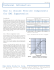### The N-wire exponential transmission line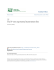### THE TONKER™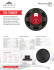### Here - Ferronics, Inc.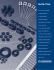### Soft Ferrites – A User`s Guide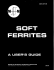### AN139 - Power Supply Layout and EMI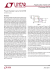### Fostex - SuonoStore.com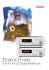### Yamaha P-2200 Manual### Geothermal Energy in the Netherlands: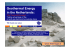### EMI PRODUCT RANGE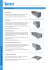### ss8.5 Brochure FA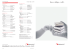### Manual - HP Archive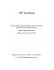### A measurement-based approach to maximum power transfer for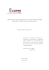### TRx115N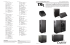### 4315 - JBL Professional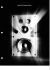### CARVIN ENGINEERING DATA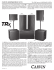### Ovnis.tv http://world.altavista.com/babelfish/trurl_pagecontent?url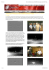### TRx122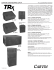### NL02-05-EN-V18 Metastudie D Vorwort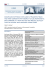### product catalog - Bienestar para Vivir